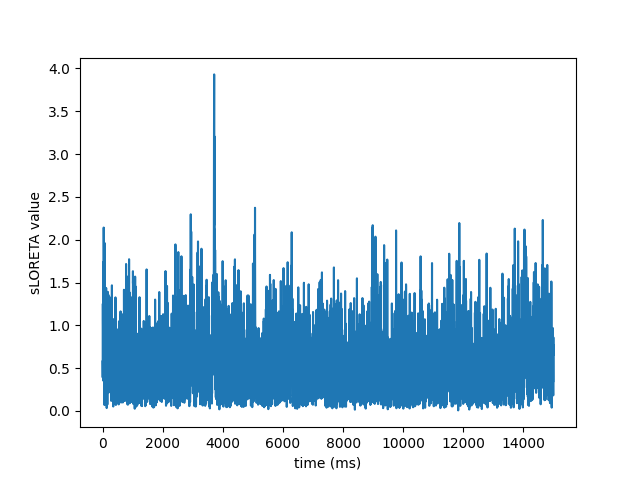# Compute sLORETA inverse solution on raw data#

Compute sLORETA inverse solution on raw dataset restricted to a brain label and stores the solution in stc files for visualisation.

```# Author: Alexandre Gramfort <alexandre.gramfort@inria.fr>
#
```
```import matplotlib.pyplot as plt

import mne
from mne.datasets import sample

print(__doc__)

data_path = sample.data_path()
fname_inv = data_path / "MEG" / "sample" / "sample_audvis-meg-oct-6-meg-inv.fif"
fname_raw = data_path / "MEG" / "sample" / "sample_audvis_raw.fif"
label_name = "Aud-lh"
fname_label = data_path / "MEG" / "sample" / "labels" / f"{label_name}.label"

snr = 1.0  # use smaller SNR for raw data
lambda2 = 1.0 / snr**2
method = "sLORETA"  # use sLORETA method (could also be MNE or dSPM)

raw.set_eeg_reference("average", projection=True)  # set average reference.
start, stop = raw.time_as_index([0, 15])  # read the first 15s of data

# Compute inverse solution
stc = apply_inverse_raw(
raw, inverse_operator, lambda2, method, label, start, stop, pick_ori=None
)

# Save result in stc files
stc.save("mne_%s_raw_inverse_%s" % (method, label_name), overwrite=True)
```
```Opening raw data file /home/circleci/mne_data/MNE-sample-data/MEG/sample/sample_audvis_raw.fif...
Read a total of 3 projection items:
PCA-v1 (1 x 102)  idle
PCA-v2 (1 x 102)  idle
PCA-v3 (1 x 102)  idle
Range : 25800 ... 192599 =     42.956 ...   320.670 secs
Reading inverse operator decomposition from /home/circleci/mne_data/MNE-sample-data/MEG/sample/sample_audvis-meg-oct-6-meg-inv.fif...
[done]
[done]
305 x 305 full covariance (kind = 1) found.
Read a total of 4 projection items:
PCA-v1 (1 x 102) active
PCA-v2 (1 x 102) active
PCA-v3 (1 x 102) active
Average EEG reference (1 x 60) active
22494 x 22494 diagonal covariance (kind = 2) found.
22494 x 22494 diagonal covariance (kind = 6) found.
22494 x 22494 diagonal covariance (kind = 5) found.
Did not find the desired covariance matrix (kind = 3)
Computing patch statistics...
[done]
Computing patch statistics...
[done]
Read a total of 4 projection items:
PCA-v1 (1 x 102) active
PCA-v2 (1 x 102) active
PCA-v3 (1 x 102) active
Average EEG reference (1 x 60) active
Source spaces transformed to the inverse solution coordinate frame
EEG channel type selected for re-referencing
1 projection items deactivated
Preparing the inverse operator for use...
Scaled noise and source covariance from nave = 1 to nave = 1
Created the regularized inverter
Created an SSP operator (subspace dimension = 3)
Created the whitener using a noise covariance matrix with rank 302 (3 small eigenvalues omitted)
Computing noise-normalization factors (sLORETA)...
[done]
Applying inverse to raw...
Picked 305 channels from the data
Computing inverse...
Eigenleads need to be weighted ...
combining the current components...
[done]
Writing STC to disk...
[done]
```

View activation time-series

```plt.plot(1e3 * stc.times, stc.data[::100, :].T)
plt.xlabel("time (ms)")
plt.ylabel("%s value" % method)
plt.show()
```Total running time of the script: (0 minutes 3.006 seconds)

Estimated memory usage: 10 MB

Gallery generated by Sphinx-Gallery